# NCERT solutions for Class 9 Maths chapter 13 - Surface Area and Volumes [Latest edition]

#### Chapters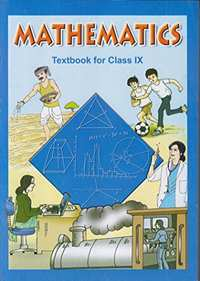## Solutions for Chapter 13: Surface Area and Volumes

Below listed, you can find solutions for Chapter 13 of CBSE NCERT for Class 9 Maths.

Exercise 13.1Exercise 13.2Exercise 13.3Exercise 13.4Exercise 13.5Exercise 13.6Exercise 13.7Exercise 13.8Exercise 13.9
Exercise 13.1 [Page 213]

### NCERT solutions for Class 9 Maths Chapter 13 Surface Area and Volumes Exercise 13.1 [Page 213]

Exercise 13.1 | Q 1 | Page 213

A plastic box 1.5 m long, 1.25 m wide and 65 cm deep, is to be made. It is to be open at the top. Ignoring the thickness of the plastic sheet, determine:

(i) The area of the sheet required for making the box.

(ii) The cost of sheet for it, if a sheet measuring 1 m2 costs Rs 20.

Exercise 13.1 | Q 2 | Page 213

The length, breadth and height of a room are 5 m, 4 m and 3 m respectively. Find the cost of white washing the walls of the room and the ceiling at the rate of Rs 7.50 per m2.

Exercise 13.1 | Q 3 | Page 213

The floor of a rectangular hall has a perimeter 250 m. If the cost of panting the four walls at the rate of Rs.10 per m2 is Rs.15000, find the height of the hall.

[Hint: Area of the four walls = Lateral surface area.]

Exercise 13.1 | Q 4 | Page 213

The paint in a certain container is sufficient to paint an area equal to 9.375 m2. How many bricks of dimensions 22.5 cm × 10 cm × 7.5 cm can be painted out of this container?

Exercise 13.1 | Q 5 | Page 213

A cubical box has each edge 10 cm and another cuboidal box is 12.5 cm long, 10 cm wide and 8 cm high.

(i) Which box has the greater lateral surface area and by how much?

(ii) Which box has the smaller total surface area and by how much?

Exercise 13.1 | Q 6.1 | Page 213

A small indoor greenhouse (herbarium) is made entirely of glass panes (including base) held together with tape. It is 30 cm long, 25 cm wide and 25 cm high. What is the area of the glass?

Exercise 13.1 | Q 6.2 | Page 213

A small indoor greenhouse (herbarium) is made entirely of glass panes (including base) held together with tape. It is 30 cm long, 25 cm wide and 25 cm high. How much of tape is needed for all the 12 edges?

Exercise 13.1 | Q 7 | Page 213

Shanti Sweets Stall was placing an order for making cardboard boxes for packing their sweets. Two sizes of boxes were required. The bigger of dimensions 25 cm × 20 cm × 5 cm and the smaller of dimensions 15 cm × 12 cm × 5 cm. For all the overlaps, 5% of the total surface area is required extra. If the cost of the cardboard is Rs 4 for 1000 cm2, find the cost of cardboard required for supplying 250 boxes of each kind.

Exercise 13.1 | Q 8 | Page 213

Parveen wanted to make a temporary shelter for her car, by making a box-like structure with tarpaulin that covers all the four sides and the top of the car (with the front face as a flap which can be rolled up). Assuming that the stitching margins are very small, and therefore negligible, how much tarpaulin would be required to make the shelter of height 2.5 m, with base dimensions 4 m × 3 m?

Exercise 13.2 [Pages 216 - 217]

### NCERT solutions for Class 9 Maths Chapter 13 Surface Area and Volumes Exercise 13.2 [Pages 216 - 217]

Exercise 13.2 | Q 1 | Page 216

The curved surface area of a right circular cylinder of height 14 cm is 88 cm2. Find the diameter of the base of the cylinder.

Assume pi = 22/7

Exercise 13.2 | Q 2 | Page 216

It is required to make a closed cylindrical tank of height 1 m and base diameter 140 cm from a metal sheet. How many square meters of the sheet are required for the same?

["Assume "pi=22/7]

Exercise 13.2 | Q 3 | Page 216

A metal pipe is 77 cm long. The inner diameter of a cross section is 4 cm, the outer diameter being 4.4 cm.(See the given figure)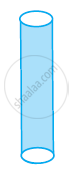(i) inner curved surface area,

(ii) outer curved surface area,

(iii) total surface area.

["Assume "pi=22/7]

Exercise 13.2 | Q 4 | Page 217

The diameter of a roller is 84 cm and its length is 120 cm. It takes 500 complete revolutions to move once over to level a playground. Find the area of the playground in m2?

["Assume "pi=22/7]

Exercise 13.2 | Q 5 | Page 217

A cylindrical pillar is 50 cm in diameter and 3.5 m in height. Find the cost of painting the curved surface of the pillar at the rate of Rs.12.50 per m2.

["Assume "pi=22/7]

Exercise 13.2 | Q 6 | Page 217

Curved surface area of a right circular cylinder is 4.4 m2. If the radius of the base of the cylinder is 0.7 m, find its height.

["Assume "pi=22/7]

Exercise 13.2 | Q 7 | Page 217

The inner diameter of a circular well is 3.5 m. It is 10 m deep. Find

(i) Its inner curved surface area,

(ii) The cost of plastering this curved surface at the rate of Rs 40 per m2.

["Assume "pi=22/7]

Exercise 13.2 | Q 8 | Page 217

In a hot water heating system, there is a cylindrical pipe of length 28 m and diameter 5 cm. Find the total radiating surface in the system.

["Assume "pi=22/7]

Exercise 13.2 | Q 9. (i) | Page 217

Find

The lateral or curved surface area of a closed cylindrical petrol storage tank that is 4.2 m in diameter and 4.5 m high.

["Assume "pi=22/7]

Exercise 13.2 | Q 9. (ii) | Page 217

Find

How much steel was actually used, if 1/12 of the steel actually used was wasted in making the tank.

["Assume "pi=22/7]

Exercise 13.2 | Q 10 | Page 217

In the given figure, you see the frame of a lampshade. It is to be covered with a decorative cloth. The frame has a base diameter of 20 cm and height of 30 cm. A margin of 2.5 cm is to be given for folding it over the top and bottom of the frame. Find how much cloth is required for covering the lampshade.

["Assume "pi=22/7]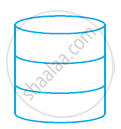Exercise 13.2 | Q 11 | Page 217

The students of a Vidyalaya were asked to participate in a competition for making and decorating penholders in the shape of a cylinder with a base, using cardboard. Each penholder was to be of radius 3 cm and height 10.5 cm. The Vidyalaya was to supply the competitors with cardboard. If there were 35 competitors, how much cardboard was required to be bought for the competition?

["Assume "pi=22/7]

Exercise 13.3 [Page 221]

### NCERT solutions for Class 9 Maths Chapter 13 Surface Area and Volumes Exercise 13.3 [Page 221]

Exercise 13.3 | Q 1 | Page 221

Diameter of the base of a cone is 10.5 cm and its slant height is 10 cm. Find its curved surface area.

["Assume "pi=22/7]

Exercise 13.3 | Q 2 | Page 221

Find the total surface area of a cone, if its slant height is 21 m and diameter of its base is 24 m.

["Assume "pi=22/7]

Exercise 13.3 | Q 3 | Page 221

Curved surface area of a cone is 308 cm2 and its slant height is 14 cm. Find

(i) radius of the base and (ii) total surface area of the cone.

["Assume "pi=22/7]

Exercise 13.3 | Q 4 | Page 221

A conical tent is 10 m high and the radius of its base is 24 m. Find

(i) slant height of the tent

(ii) cost of the canvas required to make the tent, if the cost of 1 m2 canvas is Rs 70.

["Assume "pi=22/7]

Exercise 13.3 | Q 5 | Page 221

What length of tarpaulin 3 m wide will be required to make conical tent of height 8 m and base radius 6 m? Assume that the extra length of material that will be required for stitching margins and wastage in cutting is approximately 20 cm. [Use π = 3.14]

Exercise 13.3 | Q 6 | Page 221

The slant height and base diameter of a conical tomb are 25 m and 14 m respectively. Find the cost of white-washing its curved surface at the rate of Rs 210 per 100 m2.

["Assume "pi=22/7]

Exercise 13.3 | Q 7 | Page 221

A joker’s cap is in the form of right circular cone of base radius 7 cm and height 24 cm. Find the area of the sheet required to make 10 such caps.

["Assume "pi=22/7]

Exercise 13.3 | Q 8 | Page 221

A bus stop is barricaded from the remaining part of the road, by using 50 hollow cones made of recycled cardboard. Each cone has a base diameter of 40 cm and height 1 m. If the outer side of each of the cones is to be painted and the cost of painting is Rs 12 per m2, what will be the cost of painting all these cones?

("Use "π = 3.14" and take "sqrt1.04= 1.02)

Exercise 13.4 [Page 225]

### NCERT solutions for Class 9 Maths Chapter 13 Surface Area and Volumes Exercise 13.4 [Page 225]

Exercise 13.4 | Q 1.1 | Page 225

Find the surface area of a sphere of radius 10.5 cm

["Assume "pi=22/7]

Exercise 13.4 | Q 1.2 | Page 225

Find the surface area of a sphere of radius 5.6 cm

["Assume "pi=22/7]

Exercise 13.4 | Q 1.3 | Page 225

Find the surface area of a sphere of radius 14 cm

["Assume "pi=22/7]

Exercise 13.4 | Q 2.1 | Page 225

Find the surface area of a sphere of diameter 14 cm

["Assume "pi=22/7]

Exercise 13.4 | Q 2.2 | Page 225

Find the surface area of a sphere of diameter 21 cm

["Assume "pi=22/7]

Exercise 13.4 | Q 2.3 | Page 225

Find the surface area of a sphere of diameter 3.5 m

["Assume "pi=22/7]

Exercise 13.4 | Q 3 | Page 225

Find the total surface area of a hemisphere of radius 10 cm. [Use π = 3.14]

Exercise 13.4 | Q 4 | Page 225

The radius of a spherical balloon increases from 7 cm to 14 cm as air is being pumped into it. Find the ratio of surface areas of the balloon in the two cases.

Exercise 13.4 | Q 5 | Page 225

A hemispherical bowl made of brass has inner diameter 10.5 cm. Find the cost of tin-plating it on the inside at the rate of Rs 16 per 100 cm2.

["Assume "pi=22/7]

Exercise 13.4 | Q 6 | Page 225

Find the radius of a sphere whose surface area is 154 cm2.

["Assume "pi=22/7]

Exercise 13.4 | Q 7 | Page 225

The diameter of the moon is approximately one-fourth of the diameter of the earth. Find the ratio of their surface area.

Exercise 13.4 | Q 8 | Page 225

A hemispherical bowl is made of steel, 0.25 cm thick. The inner radius of the bowl is 5 cm. Find the outer curved surface area of the bowl.

["Assume "pi=22/7]

Exercise 13.4 | Q 9 | Page 225

A right circular cylinder just encloses a sphere of radius r (see figure). Find

(i) surface area of the sphere,

(ii) curved surface area of the cylinder,

(iii) ratio of the areas obtained in (i) and (ii).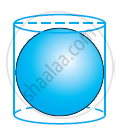Exercise 13.5 [Page 228]

### NCERT solutions for Class 9 Maths Chapter 13 Surface Area and Volumes Exercise 13.5 [Page 228]

Exercise 13.5 | Q 1 | Page 228

A matchbox measures 4 cm × 2.5 cm × 1.5 cm. What will be the volume of a packet containing 12 such boxes?

Exercise 13.5 | Q 2 | Page 228

A cuboidal water tank is 6 m long, 5 m wide and 4.5 m deep. How many litres of water can it hold? (1 m3 = 1000l)

Exercise 13.5 | Q 3 | Page 228

A cuboidal vessel is 10 m long and 8 m wide. How high must it be made to hold 380 cubic metres of a liquid?

Exercise 13.5 | Q 4 | Page 228

Find the cost of digging a cuboidal pit 8 m long, 6 m broad and 3 m deep at the rate of Rs 30 per m3.

Exercise 13.5 | Q 5 | Page 228

The capacity of a cuboidal tank is 50000 litres of water. Find the breadth of the tank, if its length and depth are respectively 2.5 m and 10 m.

Exercise 13.5 | Q 6 | Page 228

A village, having a population of 4000, requires 150 litres of water per head per day. It has a tank measuring 20 m × 15 m × 6 m. For how many days will the water of this tank last?

Exercise 13.5 | Q 7 | Page 228

A godown measures 60 m × 25 m × 15 m. Find the maximum number of wooden crates each measuring 1.5 m × 1.25 m × 0.5 m that can be stored in the godown.

Exercise 13.5 | Q 8 | Page 228

A solid cube of side 12 cm is cut into eight cubes of equal volume. What will be the side of the new cube? Also, find the ratio between their surface areas.

Exercise 13.5 | Q 9 | Page 228

A river 3 m deep and 40 m wide is flowing at the rate of 2 km per hour. How much water will fall into the sea in a minute?

Exercise 13.6 [Pages 230 - 231]

### NCERT solutions for Class 9 Maths Chapter 13 Surface Area and Volumes Exercise 13.6 [Pages 230 - 231]

Exercise 13.6 | Q 1 | Page 230

The circumference of the base of the cylindrical vessel is 132 cm and its height is 25 cm. How many litres of water can it hold? (1000 cm3 = 1l)

["Assume "pi=22/7]

Exercise 13.6 | Q 2 | Page 230

The inner diameter of a cylindrical wooden pipe is 24 cm and its outer diameter is 28 cm. The length of the pipe is 35 cm. Find the mass of the pipe, if 1 cm3 of wood has a mass of 0.6 g.

["Assume "pi=22/7]

Exercise 13.6 | Q 3 | Page 230

A soft drink is available in two packs − (i) a tin can with a rectangular base of length 5 cm and width 4 cm, having a height of 15 cm and (ii) a plastic cylinder with circular base of diameter 7 cm and height 10 cm. Which container has greater capacity and by how much?

["Assume "pi=22/7]

Exercise 13.6 | Q 4 | Page 230

If the lateral surface of a cylinder is 94.2 cm2 and its height is 5 cm, then find (i) radius of its base (ii) its volume. [Use π = 3.14]

Exercise 13.6 | Q 5 | Page 231

It costs Rs 2200 to paint the inner curved surface of a cylindrical vessel 10 m deep. If the cost of painting is at the rate of Rs 20 per m2, find

(i) Inner curved surface area of the vessel

(iii) Capacity of the vessel

["Assume "pi=22/7]

Exercise 13.6 | Q 6 | Page 231

The capacity of a closed cylindrical vessel of height 1 m is 15.4 litres. How many square metres of metal sheet would be needed to make it?

["Assume "pi=22/7]

Exercise 13.6 | Q 7 | Page 231

A lead pencil consists of a cylinder of wood with solid cylinder of graphite filled in the interior. The diameter of the pencil is 7 mm and the diameter of the graphite is 1 mm. If the length of the pencil is 14 cm, find the volume of the wood and that of the graphite.

["Assume "pi=22/7]

Exercise 13.6 | Q 8 | Page 231

A patient in a hospital is given soup daily in a cylindrical bowl of diameter 7 cm. If the bowl is filled with soup to a height of 4 cm, how much soup the hospital has to prepare daily to serve 250 patients?

["Assume "pi=22/7]

Exercise 13.7 [Page 233]

### NCERT solutions for Class 9 Maths Chapter 13 Surface Area and Volumes Exercise 13.7 [Page 233]

Exercise 13.7 | Q 1.1 | Page 233

Find the volume of the right circular cone with radius 6 cm and height 7 cm

["Assume "pi=22/7]

Exercise 13.7 | Q 1.2 | Page 233

Find the volume of the right circular cone with radius 3.5 cm, height 12 cm

["Assume "pi=22/7]

Exercise 13.7 | Q 2.1 | Page 233

Find the capacity in litres of a conical vessel with radius 7 cm, slant height 25 cm

["Assume "pi=22/7]

Exercise 13.7 | Q 2.2 | Page 233

Find the capacity in litres of a conical vessel with height 12 cm, slant height 13 cm

["Assume "pi=22/7]

Exercise 13.7 | Q 3 | Page 233

The height of a cone is 15 cm. If its volume is 1570 cm3, find the diameter of its base. [Use π = 3.14]

Exercise 13.7 | Q 4 | Page 233

If the volume of a right circular cone of height 9 cm is 48π cm3, find the diameter of its base.

Exercise 13.7 | Q 5 | Page 233

A conical pit of top diameter 3.5 m is 12 m deep. What is its capacity in kilolitres?

["Assume "pi=22/7]

Exercise 13.7 | Q 6 | Page 233

The volume of a right circular cone is 9856 cm3. If the diameter of the base is 28 cm, find

(i) height of the cone

(ii) slant height of the cone

(iii) curved surface area of the cone

["Assume "pi=22/7]

Exercise 13.7 | Q 7 | Page 233

A right triangle ABC with sides 5 cm, 12 cm and 13 cm is revolved about the side 12 cm. Find the volume of the solid so obtained.

Exercise 13.7 | Q 8 | Page 233

A right triangle ABC with sides 5 cm, 12 cm and 13 cm is revolved about the side 12 cm. Find the volume of the solid so obtained.

If the triangle ABC in the above Question is revolved about the side 5 cm, then find the volume of the solid so obtained. Find also the ratio of the volumes of the two solids obtained.

Exercise 13.7 | Q 9 | Page 233

A heap of wheat is in the form of a cone whose diameter is 10.5 m and height is 3 m. Find its volume. The heap is to be covered by canvas to protect it from rain. Find the area of the canvas required.

["Assume "pi=22/7]

Exercise 13.8 [Page 236]

### NCERT solutions for Class 9 Maths Chapter 13 Surface Area and Volumes Exercise 13.8 [Page 236]

Exercise 13.8 | Q 1.1 | Page 236

Find the volume of a sphere whose radius is 7 cm.

["Assume "pi=22/7]

Exercise 13.8 | Q 1.2 | Page 236

Find the volume of a sphere whose radius is 0.63 m

["Assume "pi=22/7]

Exercise 13.8 | Q 2.1 | Page 236

Find the amount of water displaced by a solid spherical ball of diameter 28 cm.

["Assume "pi=22/7]

Exercise 13.8 | Q 2.2 | Page 236

Find the amount of water displaced by a solid spherical ball of diameter 0.21 m.

["Assume "pi=22/7]

Exercise 13.8 | Q 3 | Page 236

The diameter of a metallic ball is 4.2 cm. What is the mass of the ball, if the density of the metal is 8.9 g per cm3?

["Assume "pi=22/7]

Exercise 13.8 | Q 4 | Page 236

The diameter of the moon is approximately one-fourth of the diameter of the earth. What fraction of the volume of the earth is the volume of the moon?

Exercise 13.8 | Q 5 | Page 236

How many litres of milk can a hemispherical bowl of diameter 10.5 cm hold?

["Assume "pi=22/7]

Exercise 13.8 | Q 6 | Page 236

A hemispherical tank is made up of an iron sheet 1 cm thick. If the inner radius is 1 m, then find the volume of the iron used to make the tank.

["Assume "pi=22/7]

Exercise 13.8 | Q 7 | Page 236

Find the volume of a sphere whose surface area is 154 cm2.

["Assume "pi=22/7]

Exercise 13.8 | Q 8 | Page 236

A dome of a building is in the form of a hemisphere. From inside, it was white-washed at the cost of Rs 498.96. If the cost of white-washing is Rs 2.00 per square meter, find the

(i) inside surface area of the dome,

(ii) volume of the air inside the dome.

["Assume "pi=22/7]

Exercise 13.8 | Q 9 | Page 236

Twenty seven solid iron spheres, each of radius r and surface area S are melted to form a sphere with surface area S'. Find the

(i) radius r' of the new sphere, (ii) ratio of S and S'.

Exercise 13.8 | Q 10 | Page 236

A capsule of medicine is in the shape of a sphere of diameter 3.5 mm. How much medicine (in mm3) is needed to fill this capsule?

["Assume "pi=22/7]

Exercise 13.9 [Pages 236 - 237]

### NCERT solutions for Class 9 Maths Chapter 13 Surface Area and Volumes Exercise 13.9 [Pages 236 - 237]

Exercise 13.9 | Q 1 | Page 236

A wooden bookshelf has external dimensions as follows: Height = 110 cm, Depth = 25 cm, Breadth = 85 cm (see the given figure). The thickness of the plank is 5 cm everywhere. The external faces are to be polished and the inner faces are to be painted. If the rate of polishing is 20 paise per cm2 and the rate of painting is 10 paise per cm2, find the total expenses required for polishing and painting the surface of the bookshelf.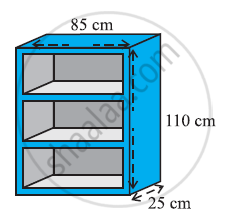Exercise 13.9 | Q 2 | Page 237

The front compound wall of a house is decorated by wooden spheres of diameter 21 cm, placed on small supports as shown in the given figure. Eight such spheres are used for this purpose, and are to be painted silver. Each support is a cylinder of radius 1.5 cm and height 7 cm and is to be painted black. Find the cost of paint required if silver paint costs 25 paise per cm2 and black paint costs 5 paise per cm2.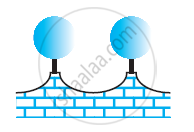Exercise 13.9 | Q 3 | Page 237

The diameter of a sphere is decreased by 25%. By what per cent does its curved surface area decrease?

## Solutions for Chapter 13: Surface Area and Volumes

Exercise 13.1Exercise 13.2Exercise 13.3Exercise 13.4Exercise 13.5Exercise 13.6Exercise 13.7Exercise 13.8Exercise 13.9## NCERT solutions for Class 9 Maths chapter 13 - Surface Area and Volumes

Shaalaa.com has the CBSE Mathematics Class 9 Maths CBSE solutions in a manner that help students grasp basic concepts better and faster. The detailed, step-by-step solutions will help you understand the concepts better and clarify any confusion. NCERT solutions for Mathematics Class 9 Maths CBSE 13 (Surface Area and Volumes) include all questions with answers and detailed explanations. This will clear students' doubts about questions and improve their application skills while preparing for board exams.

Further, we at Shaalaa.com provide such solutions so students can prepare for written exams. NCERT textbook solutions can be a core help for self-study and provide excellent self-help guidance for students.

Concepts covered in Class 9 Maths chapter 13 Surface Area and Volumes are Surface Area of a Cube, Surface Area of a Cuboid, Surface Area of Cylinder, Surface Area of a Right Circular Cone, Surface Area of a Sphere, Volume of a Cuboid, Volume of a Cylinder, Volume of a Right Circular Cone, Volume of a Sphere.

Using NCERT Class 9 Maths solutions Surface Area and Volumes exercise by students is an easy way to prepare for the exams, as they involve solutions arranged chapter-wise and also page-wise. The questions involved in NCERT Solutions are essential questions that can be asked in the final exam. Maximum CBSE Class 9 Maths students prefer NCERT Textbook Solutions to score more in exams.

Get the free view of Chapter 13, Surface Area and Volumes Class 9 Maths additional questions for Mathematics Class 9 Maths CBSE, and you can use Shaalaa.com to keep it handy for your exam preparation.